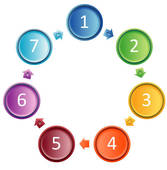Arithmetic Sequences
3 years ago
nfiorletti
Save
Edit
Host a game
Live GameLive
Homework
Solo Practice
Practice16 QuestionsShow answers
• Question 1
120 seconds
Q. Is the sequence arithmetic: 37, 31, 25, 19, ...
Yes
No
• Question 2
120 seconds
Q. Is the sequence arithmetic: -3, 3, -3, 3, ...
Yes
No
• Question 3
120 seconds
Q. Is the sequence arithmetic: 78, 785, 7855, 78555, ...
Yes
No
• Question 4
120 seconds
Q. Is the sequence arithmetic: 17, 9, 1, -7, ...
Yes
No
• Question 5
180 seconds
Q. Find the common difference: -34, -29, -24, -19, ...
d = -5
d = 5
d = -6
d = 6
• Question 6
180 seconds
Q. Find the common difference: 29, 21, 13, 5, ...
d = -10
d = -9
d = -8
d = -7
• Question 7
180 seconds
Q. Find the common difference: -18, -23, -28, -33, ...
d = -5
d = 5
d = -3
d = 3
• Question 8
180 seconds
Q. Find the common difference: 1, 201, 401, 601, ...
d = 100
d = 101
d = 200
d = 201
• Question 9
300 seconds
Q. Find the next three terms in the arithmetic sequence: -33, -24, -15, -6, ...
-1, 7, 15
-5, 2, 9
3, 12, 21
-65, -73, -81
• Question 10
300 seconds
Q. Find the next three terms in the arithmetic sequence: -3, -8, -13, -18, ...
-20, -24, -28
-21, -25, -29
-23, -28, -33
-24, -29, -32
• Question 11
300 seconds
Q. Find the next three terms in the arithmetic sequence: 9, 19, 29, 39, ...
45, 54, 63
49, 59, 69
39, 49, 59
59, 69, 79
• Question 12
300 seconds
Q. Find the next three terms in the arithmetic sequence: 25, 34, 43, 52, ...
63, 72, 81
79, 88, 97
70, 79, 88
61, 70, 79
• Question 13
300 seconds
Q. Find the 35th term in the arithmetic sequence: -10, -14, -18, -22, ...
-216
-148
-146
-150
• Question 14
300 seconds
Q. Find a26 in the arithmetic sequence: -15, -35, -55, -75, ...
-515
-490
-535
460
• Question 15
300 seconds
Q. Find the 26th term in the arithmetic sequence: 20, 26, 32, 38, ...
182
176
170
-118
• Question 16
300 seconds
Q. Find a20 in the arithmetic sequence: 5, 12, 19, 26...